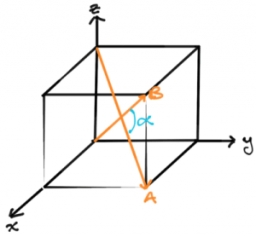# Angle of the body diagonals

Using vector dot product calculate the angle of the body diagonals of the cube.

A =  70.5288 °

### Step-by-step explanation:Did you find an error or inaccuracy? Feel free to write us. Thank you!Tips to related online calculators
Line slope calculator is helpful for basic calculations in analytic geometry. The coordinates of two points in the plane calculate slope, normal and parametric line equation(s), slope, directional angle, direction vector, the length of the segment, intersections of the coordinate axes, etc.
Our vector sum calculator can add two vectors given by their magnitudes and by included angle.
Pythagorean theorem is the base for the right triangle calculator.

#### You need to know the following knowledge to solve this word math problem:

We encourage you to watch this tutorial video on this math problem:

## Related math problems and questions:

• Space vectors 3DThe vectors u = (1; 3; -4), v = (0; 1; 1) are given. Find the size of these vectors, calculate the angle of the vectors, the distance between the vectors.
• TrianglePlane coordinates of vertices: K[11, -10] L[10, 12] M[1, 3] give Triangle KLM. Calculate its area and its interior angles.
• Angle between vectorsFind the angle between the given vectors to the nearest tenth of a degree. u = (-22, 11) and v = (16, 20)
• Scalar dot productCalculate u.v if |u| = 5, |v| = 2 and when angle between the vectors u, v is: a) 60° b) 45° c) 120°
• CuboidsTwo separate cuboids with different orientation in space. Determine the angle between them, knowing the direction cosine matrix for each separate cuboid. u1=(0.62955056, 0.094432584, 0.77119944) u2=(0.14484653, 0.9208101, 0.36211633)
• Cube - anglesCalculate angle between the wall diagonal and cube base. Calculate the angle between the cube body diagonal and cube base.
• Space diagonal anglesCalculate the angle between the body diagonal and the side edge c of the block with dimensions: a = 28cm, b = 45cm and c = 73cm. Then, find the angle between the body diagonal and the plane of the base ABCD.
• VectorCalculate length of the vector v⃗ = (9.75, 6.75, -6.5, -3.75, 2).
• BoxCalculate the angle between box base 9 x 14 and body diagonal length 18.
• Diagonals of a prismThe base of the square prism is a rectangle with dimensions of 3 dm and 4 dm. The height of the prism is 1 m. Find out the angle between the body diagonal with the diagonal of the base.
• Vector sumThe magnitude of the vector u is 12 and the magnitude of the vector v is 8. Angle between vectors is 61°. What is the magnitude of the vector u + v?
• ForcesIn point O acts three orthogonal forces: F1 = 20 N, F2 = 7 N, and F3 = 19 N. Determine the resultant of F and the angles between F and forces F1, F2, and F3.
• DiagonalDetermine the dimensions of the cuboid, if diagonal long 53 dm has an angle with one edge 42° and with another edge 64°.
• Decide 2Decide whether points A[-2, -5], B[4, 3] and C[16, -1] lie on the same line
• Body diagonalCalculate the cube volume, whose body diagonal size is 75 dm. Draw a picture and highlight the body diagonal.
• Cube diagonalsDetermine the volume and surface area of the cube if you know the length of the body diagonal u = 216 cm.
• Body diagonalCalculate the length of the body diagonal of the 6cm cube.